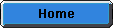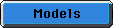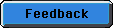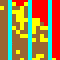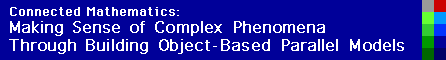Laplace - Heat Flow

The Heat Flow models allow us to conceptualize heat flow on a thin plate where one is able to describe the exact temperatures on each side and then chart the process of the temperature distribution in real time. There are currently three Heat Flow models. Laplace is the simplest; it fixes the temperature of each edge of the plate and finds the temperature on each part of the plate when it reaches equilibrium. The Poisson model is similar but adds a heat source or sink to any point on the plate. The Diffusion model is like Laplace except that it is also "time-accurate"; that is, it simulates the actual process of gradual heat transfer through the plate over time.

For detailed instructions on using the program, take a look at the info window from the Laplace - Heat Flow model.

Download the StarLogoT source code for the Laplace - Heat Flow model: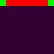In this model the temperature of the top of the plate was 999 and the temperature of the left, right, and bottom sides of the plate was 0. (57 k)